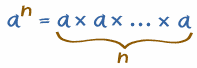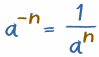# Exponents

The exponent of a number says how many times to use the number in a multiplication.In 82 the "2" says to use 8 twice in a multiplication,
so 82 = 8 × 8 = 64

In words: 82 could be called "8 to the power 2" or "8 to the second power", or simply "8 squared"

Some more examples:

### Example: 53 = 5 × 5 × 5 = 125

• In words: 53 could be called "5 to the third power", "5 to the power 3" or simply "5 cubed"

### Example: 24 = 2 × 2 × 2 × 2 = 16

• In words: 24 could be called "2 to the fourth power" or "2 to the power 4" or simply "2 to the 4th"

Exponents make it easier to write and use many multiplications

Example: 96 is easier to write and read than 9 × 9 × 9 × 9 × 9 × 9

Note: Exponents are also called Powers or Indices.

You can multiply any number by itself
as many times as you want using exponents.

Try here:

algebra/images/exponent-calc.js

So in general:

 an tells you to multiply a by itself, so there are n of those a's:## Another Way of Writing It

Sometimes people use the ^ symbol (above the 6 on your keyboard), as it is easy to type.

Example: 2^4 is the same as 24

• 2^4 = 2 × 2 × 2 × 2 = 16

## Negative Exponents

Negative? What could be the opposite of multiplying? Dividing!

So we divide by the number each time, which is the same as multiplying by 1number

Example: 8-1 = 18 = 0.125

We can continue on like this:

Example: 5-3 = 15 × 15 × 15 = 0.008

But it is often easier to do it this way:

5-3 could also be calculated like:

15 × 5 × 5 = 153 = 1125 = 0.008

## Negative? Flip the Positive!That last example showed an easier way to handle negative exponents: Calculate the positive exponent (an) Then take the Reciprocal (i.e. 1/an)

More Examples:

Negative Exponent   Reciprocal of
Positive Exponent
4-2 = 1 / 42 = 1/16 = 0.0625
10-3 = 1 / 103 = 1/1,000 = 0.001
(-2)-3 = 1 / (-2)3 = 1/(-8) = -0.125

## What if the Exponent is 1, or 0?

 1 If the exponent is 1, then you just have the number itself (example 91 = 9) 0 If the exponent is 0, then you get 1 (example 90 = 1) But what about 00 ? It could be either 1 or 0, and so people say it is "indeterminate".

## It All Makes Sense

If you look at that table, you will see that positive, zero or negative exponents are really part of the same (fairly simple) pattern:

Example: Powers of 5
.. etc..52 5 × 5 25
51 5 5
50 1 1
5-1 15 0.2
5-2 15 × 15 0.04
.. etc..